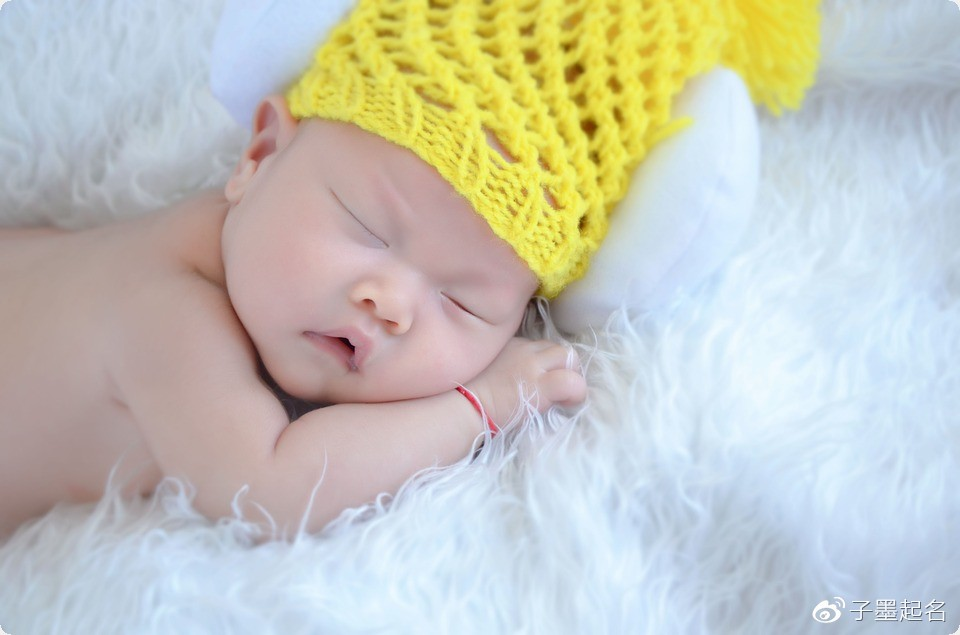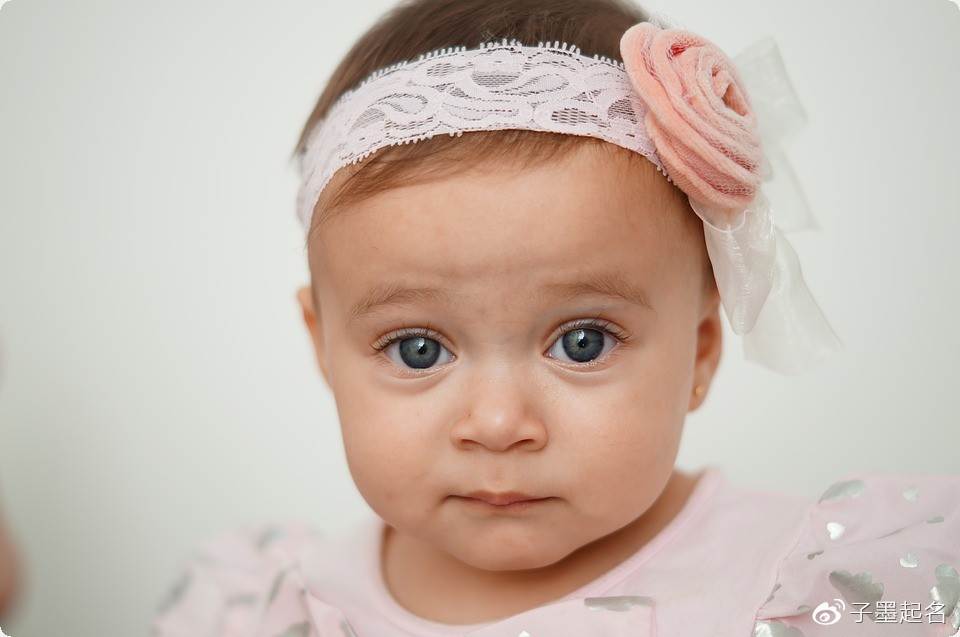﻿ 600个狗年女宝宝取名专用字，让您再也不愁没有好名字-尊享起名网（1）惠+慧+敏+旭+春+晴+曼+月+姬+娅+娆+娇

（2）娉+娜+娟+琳+琴+琼+咏+卿+澜+琴+纨+仪

（3）娣+娥+娴+婉+婕+婧+婵+婷+琦+琪+琬+琰

（4）瑗+瑛+瑜+瑶+毓+悦+枫+芸+菲+融+园+艺

（5）青+倩+婷+姣+婉+娴+瑾+晓+欢+蓉+眉+君

（6）荷+丹+蕊+薇+菁+梦+瑶+怡+真+环+雪+荣

（7）爱+妹+月+琦+晶+妍+瑾+颖+露+薇+菁+梦

（8）莺+媛+艳+瑞+凡+佳+嘉+琼+叶+璧+璐+娅

（9）茜+秋+珊+莎+锦+黛+青+倩+婷+姣+婉+娴

（10）瑶+怡+婵+雁+仪+荷+丹+蓉+眉+君+琴+蕊

（11）岚+苑+婕+馨+融+园+艺+咏+卿+聪+澜+纯

（12）夕+月+悦+萱+娅+媛+翠+雅+芝+伊+英+玉

（13）乐+彤+影+珍+依+沫+玉+灵+瑶+嫣+倩+妍

（14）怡+佩+淇+雨+娜+莹+娟+文+芳+莉+丽+美

（15）文+晨+宇+怡+全+子+凡+悦+思+贞+荣+琼

（16）筠+柔+竹+霭+凝+晓+欢+霄+枫+芸+菲+寒

（17）亚+宜+可+姬+舒+影+荔+枝+思+丽+秀+娟

（18）华+慧+巧+美+娜+静+淑+惠+珠+翠+雅+芝

（19）萍+红+娥+玲+芬+芳+燕+彩+春+菊+勤+珍

（20）莉+兰+凤+洁+梅+琳+素+云+莲+真+环+雪（21）爱+妹+霞+香+月+莺+媛+艳+瑞+凡+佳+嘉

（22）桂+娣+叶+璧+璐+娅+琦+晶+妍+茜+秋+珊

（23）莎+怡+薇+咏+希+寒+锦+雁+岚+澜+育+芸

（24）锦+黛+青+倩+婷+姣+婉+娴+瑾+颖+露+瑶

（25）婵+雁+蓓+纨+仪+荷+丹+蓉+眉+君+琴+蕊

（26）菁+梦+岚+苑+婕+馨+瑗+琰+韵+融+园+艺

（27）卿+聪+澜+纯+毓+悦+昭+冰+爽+琬+茗+羽

（28）宁+欣+飘+育+滢+馥+伊+亚+宜+可+柔+菲

（29）叶+璐+娅+琦+晶+妍+瑶+毓+茜+秋+珊+莎

（30）黛+青+倩+婷+姣+婉+娴+瑾+颖+露+怡+婵

（31）蓓+纨+仪+荷+丹+蓉+眉+君+琴+蕊+薇+菁

（32）苑+婕+馨+瑗+琰+韵+融+园+艺+咏+卿+聪

（33）纯+悦+昭+冰+爽+琬+茗+羽+希+宁+欣+飘

（34）滢+馥+筠+竹+霭+凝+晓+欢+霄+枫+芸+沫

（35）涵+筠+夕+柔+乐+竹+莹+霭+凝+晗+枫+依

（36）晓+欢+晴+霄+彤+菲+灵+寒+燕+嫣+彩+华

（37）玲+芬+苑+婕+馨+蝶+琳+素+妍+萱+慧+菱（38）佩+伊+清+亚+海+宜+雯+可+梓+巧+嘉+蔓

（39）晨+美+琪+娜+茹+静+吉+芳+榕+妹+洋+昭

（40）雨+姬+子+舒+钰+影+诗+金+枝+若+熙+凛

（41）思+丽+敏+秀+奕+娟+璇+英+楚+珺+沛+洺

（42）文+淑+萌+惠+如+珠+紫+翠+小+蓓+学+语

（43）一+雅+心+芝+玉+萍+米+红+昕+炜+苓+翎

（44）春+妙+云+莲+真+环+雪+荣+爱+筱+潇+玥

（45）珊+莎+锦+黛+青+倩+婷+姣+岚+霖+茜+秋

（46）菊+霜+勤+烟+珍+蕾+贞+曼+莉+薇+菁+梦

（47）兰+双+凤+芙+洁+梅+宛+绮+慕+育+滢+馥

（48）霞+香+月+莺+媛+艳+瑞+凡+佳+明+瑗+琰

（49）琼+桂+娣+叶+璧+璐+娅+琦+晶+韵+融+淼

（50）婉+娴+瑾+颖+露+瑶+怡+婵+雁+菡+圆+幼

（51）纨+仪+荷+丹+蓉+眉+君+琴+蕊+曦+希+宁

（52）园+艺+咏+卿+聪+澜+纯+毓+悦+欣+飘+羽